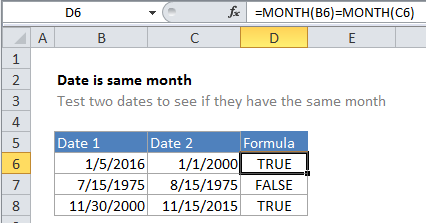## Excel Office

Excel How Tos, Tutorials, Tips & Tricks, Shortcuts

# Check If Two Dates are same month in Excel

This tutorial shows how to compare dates in Excel.

To test two dates to see they both have the same month, you can do so with a simple formula that uses the MONTH function.

## Formula

`=MONTH(date1)=MONTH(date2)`## Explanation

In the example shown, the formula in cell D6 is:

`=MONTH(B6)=MONTH(C6)`

## How the formula works

In this case, Excel extracts the month from the date in cell B6 as a number, and the month in the cell C6 as a number, then tests for equivalency using the equal sign. Both dates are in January, so the formula is solved as follows and returns TRUE.

```=MONTH(B6)=MONTH(C6)
=1=1
=TRUE```

### Same month and year

To test that a date is the same month and year is another date, you can use this clever formula proposed by reader Eric Kalin:

`EOMONTH(date1,0)=EOMONTH(date2,0)`

### Same month as today

If you need to test a date to see if has the same month as the current date (today), you can use this formula:

`=MONTH(date)=MONTH(TODAY())`
Worked Example:   MONTH function: Description, Usage, Syntax, Examples and Explanation